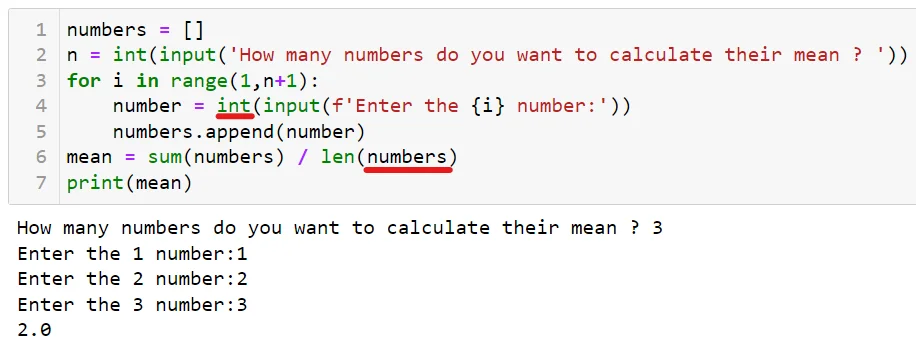21 Jul 2023

Posted on:

20 Jul 2023

0

# What's wrong with my code Q5

numbers = []
n = int(input('How many numbers do you want to calculate their mean ? '))
for i in range(1,n+1):
number = print(input(f'Enter the {i} number:'))
numbers.append(number)
mean = sum(numbers) / len(number)
print(mean)

Instructor
Posted on:

21 Jul 2023

0

Hey Ahmed,

Thank you for reaching out!

Study the following screenshot:The first thing I've changed in your code is to substitute the `print()` function with an `int()`. This converts the user's input into an integer that you can then append to your `numbers` list.

Second, I've substituted `len(number)` with `len(numbers)` as the variable `number` is the user's input while the variable `numbers` stores all inputs.

Kind regards,

365 Hristina